Home Practice
For learners and parents For teachers and schools
Textbooks
Full catalogue
Pricing SupportLog in

We think you are located in United States. Is this correct?

# 14.4 The fertiliser industry

## 14.4 The fertiliser industry (ESCS3)

### The industrial production of fertilisers (ESCS4)

This section is content heavy and an important sub-section in this chapter. It should be linked to rate of reactions, equilibrium and acid-base reactions done earlier in Grade 12.

Two lessons are allocated, but if three are available it would be advisable to spend this extra time on this sub-section (although the depth of information provided here is not required from the learners). For this section a poster, an animation and accompanying worksheet are available. These can be used as an introduction to the section as well as a classwork activity. Play the animation once or twice without the learners writing anything, then hand out the worksheet, and play the animation again so that learners can complete the worksheet.

For more information on this section refer to the Chemical Industries Resource Pack.

The industrial production of fertilisers involves several processes. Figure 14.3 summarises how a number of different industrial processes are used to manufacture a variety of fertilisers. In the following sections we will discuss the various processes indicated on this diagram.

### Producing hydrogen: Coal gasification and steam reforming at Sasol (ESCS5)

Sasol is an international company that was founded in Sasolburg, South Africa, in 1950. It employs over $$\text{34 000}$$ people in at least $$\text{38}$$ countries and has interests in synthetic fuels, mining, oil, gas and chemistry.

Fossil fuels are the main source of industrial hydrogen. Hydrogen can be generated from natural gas or coal. These processes are used by Sasol at their Gas-to-Liquid (GTL) and Coal-to-Liquid (CTL) facilities. Hydrogen is usually produced by the steam reforming of methane gas (natural gas). At high temperatures ($$\text{700}$$ – $$\text{1 100}$$$$\text{°C}$$), steam ($$\text{H}_{2}\text{O}$$) reacts with methane ($$\text{CH}_{4}$$) in an endothermic reaction to yield syngas, a mixture of carbon monoxide ($$\text{CO}$$) and hydrogen ($$\text{H}_{2}$$).

You are not required to know this information in as much depth as is provided here, but the extra information should help your understanding of the subject.

$$\text{CH}_{4}(\text{g}) + \text{H}_{2}\text{O}(\text{g})$$ $$\to$$ $$\text{CO}(\text{g}) + 3\text{H}_{2}(\text{g})$$

During a second stage, which takes place at a lower temperature of about $$\text{130}$$$$\text{°C}$$, the exothermic reaction generates additional hydrogen. This is called a water gas shift reaction.

$$\text{CO}(\text{g}) + \text{H}_{2}\text{O}(\text{g})$$ $$\to$$ $$\text{CO}_{2}(\text{g}) + \text{H}_{2}(\text{g})$$

Essentially, the oxygen (O) atom is stripped from the additional water (steam) to oxidise $$\text{CO}$$ to $$\text{CO}_{2}$$. This oxidation also provides energy to maintain the reaction.

Coal can also be used to produce syngas in a similar way to natural gas. The reactions are shown below:

$$\text{C}(\text{s}) + \text{H}_{2}\text{O}(\text{g})$$ $$\to$$ $$\text{CO}(\text{g}) + \text{H}_{2}(\text{g})$$

$$\text{CO}(\text{g}) + \text{H}_{2}\text{O}(\text{g})$$ $$\to$$ $$\text{CO}_{2}(\text{g}) + \text{H}_{2}(\text{g})$$

Remember that yield describes the quantity of product in a container relative to the maximum product possible.

### Obtaining nitrogen: Fractional distillation of liquefied air (ESCS6)

Fractional distillation is a separation method. It uses the difference in boiling temperatures of the components of a mixture to separate those components.

• The mixture is heated to convert the components into the vapour (gas) phase.

• The vapour mixture is then pumped into a tall separation column (called a fractional distillation column), usually at the bottom of the column.

• As the vapour mixture moves up the column and cools, the different components (called fractions) condense as the temperature drops below the various boiling point temperatures.

• These fractions are collected using collection trays.

• The fractions can be removed from the mixture in this way and thus the components are separated.

This process is used to separate the components of air or crude oil. Air is a mixture of gases, mainly nitrogen ($$\text{N}_{2}$$) and oxygen ($$\text{O}_{2}$$). Liquefied air (compressed and cooled to $$-\text{200}$$$$\text{°C}$$) is pumped into the fractional distillation column. Nitrogen gas has the lowest boiling point temperature and is collected at the top of the column (Figure 14.4).

### Producing ammonia: The Haber process (ESCS7)

Ammonia ($$\text{NH}_{3}$$) plays an important role in the manufacturing process of fertilisers. The industrial process used to produce ammonia is called the Haber process. In this reaction nitrogen gas and hydrogen gas react to produce ammonia gas. The equation for the Haber process is:

$$\text{N}_{2}(\text{g}) + 3\text{H}_{2}(\text{g})$$ $$\rightleftharpoons$$ $$2\text{NH}_{3}(\text{g})$$

This reaction takes place in the presence of an iron (Fe) catalyst under high pressure ($$\text{200}$$ atmospheres (atm)) and temperature ($$\text{450}$$ – $$\text{500}$$$$\text{°C}$$) conditions.

$$\text{1}$$ $$\text{atm}$$ = $$\text{101,3}$$ $$\text{kPa}$$

By the $$\text{20}$$th century, a number of methods had been developed to fix atmospheric nitrogen, in other words to make it usable for plants. Sources of nitrogen for fertilisers included saltpetre ($$\text{NaNO}_{3}$$) from Chile, and guano. Guano is the droppings of seabirds, bats and also seals. In the early $$\text{20}$$th century, before the start of the First World War, the Haber process was developed by two German chemists, Fritz Haber and Karl Bosch. They determined what the best conditions were in order to get a high yield of ammonia, and found these to be high temperature and high pressure. During World War I, the ammonia produced by the Haber process was used to make explosives.

The forward reaction of the Haber process is exothermic, so the forward reaction is favoured by low temperatures. However, low temperatures slow all chemical reactions. So, the Haber process requires high temperatures, and the ammonia is removed as soon as it is formed to prevent it being used in the reverse reaction.

temp text

### Producing nitric acid: The Ostwald process (ESCS8)

The Ostwald process is used to produce nitric acid from ammonia. Nitric acid can then be used in reactions that produce fertilisers. Ammonia is converted to nitric acid in a three-step process.

Firstly ammonia is oxidised by heating it with oxygen, in the presence of a platinum ($$\text{Pt}$$) catalyst, to form nitrogen monoxide ($$\text{NO}$$) and water. This step is strongly exothermic, which makes it a useful heat source. The reaction that takes place is:

$$4\text{NH}_{3}(\text{g}) + 5\text{O}_{2}(\text{g})$$ $$\to$$ $$4\text{NO}(\text{g}) + 6\text{H}_{2}\text{O}(\text{g})$$

In the second step, nitrogen monoxide is oxidised again to yield nitrogen dioxide ($$\text{NO}_{2}$$) according to the following reaction:

$$2\text{NO}(\text{g}) + \text{O}_{2}(\text{g})$$ $$\to$$ $$2\text{NO}_{2}(\text{g})$$

In step three, nitrogen dioxide is absorbed by water to produce nitric acid ($$\text{HNO}_{3}$$) as follows:

$$3\text{NO}_{2}(\text{g}) + \text{H}_{2}\text{O}(\text{ℓ})$$ $$\to$$ $$2\text{HNO}_{3}(\text{aq}) + \text{NO}(\text{g})$$

Nitrogen monoxide, also known as nitrogen oxide or nitric oxide, is a by-product of this reaction. The nitrogen monoxide is recycled and the acid is concentrated to the required strength (e.g., for use in further chemical processes).

temp text

### Producing ammonium nitrate (ESCS9)

Nitric acid and ammonia can react together in an acid-base process to form the salt, ammonium nitrate ($$\text{NH}_{4}\text{NO}_{3}$$). Ammonium nitrate is soluble in water and is often used in fertilisers. The reaction is as follows:

$$\text{NH}_{3}(\text{ℓ}) + \text{HNO}_{3}(\text{ℓ})$$ $$\to$$ $$\text{NH}_{4}\text{NO}_{3}(\text{s})$$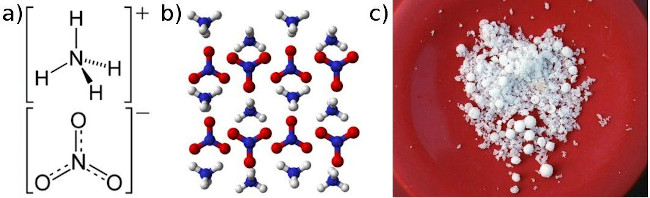Figure 14.5: Ammonium nitrate presented as a) the structural formula, b) a three-dimensional molecular representation and c) solid crystals.

### Producing urea (ESCSB)

Urea ($$(\text{NH}_{2})_{2}\text{CO}$$) is a nitrogen-containing compound that is produced on a large scale worldwide. It has the highest nitrogen content (46,4%) of all solid nitrogen-containing fertilisers that are commonly used, and is produced by the reaction of ammonia with carbon dioxide. Two reactions are involved in producing urea and ammonium carbamate ($$\text{H}_{2}\text{NCOONH}_{4}$$) is an intermediate product.

$$2\text{NH}_{3}(\text{g}) + \text{CO}_{2}(\text{g})$$ $$\rightleftharpoons$$ $$\text{H}_{2}\text{NCOONH}_{4}(\text{s})$$

$$\text{H}_{2}\text{NCOONH}_{4}(\text{s})$$ $$\rightleftharpoons$$ $$(\text{NH}_{2})_{2}\text{CO}(\text{aq}) + \text{H}_{2}\text{O}(\text{ℓ})$$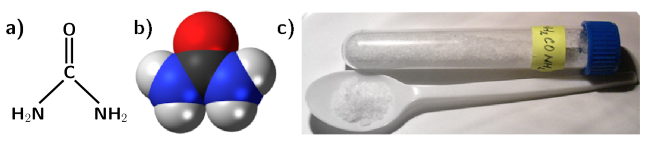Figure 14.6: Urea presented as a) the structural formula, b) a space-filling three-dimensional model and c) solid crystals.

In 1828, the German chemist Friedrich Wöhler obtained urea by treating silver cyanate ($$\text{AgOCN}$$) with ammonium chloride ($$\text{NH}_{4}\text{Cl}$$):

$$\text{AgOCN} + \text{NH}_{4}\text{Cl}$$ $$\to$$ $$(\text{NH}_{2})_{2}\text{CO} + \text{AgCl}$$

This was the first time an organic compound was artificially synthesised from inorganic starting materials, without the use of living organisms.

### Producing sulfuric acid: The Contact process (ESCSC)

Sulfuric acid ($$\text{H}_{2}\text{SO}_{4}$$) is produced from sulfur, oxygen and water through the Contact process. In the first step, sulfur is burned to produce sulfur dioxide ($$\text{SO}_{2}$$):

$$\text{S}(\text{s}) + \text{O}_{2}(\text{g})$$ $$\to$$ $$\text{SO}_{2}(\text{g})$$

This is then oxidised to sulfur trioxide ($$\text{SO}_{3}$$) using oxygen in the presence of a vanadium(V) oxide catalyst:

$$2\text{SO}_{2}(\text{g}) + \text{O}_{2}(\text{g})$$ $$\rightleftharpoons$$ $$2\text{SO}_{3}(\text{g})$$

Finally the sulfur trioxide is treated with water to produce sulfuric acid with a purity of $$\text{98}$$ - 99%:

$$\text{SO}_{3}(\text{g}) + \text{H}_{2}\text{O}(\text{ℓ})$$ $$\to$$ $$\text{H}_{2}\text{SO}_{4}(\text{ℓ})$$

temp text

### Producing ammonium sulfate (ESCSD)

Ammonium sulfate ($$(\text{NH}_{4})_{2}\text{SO}_{4}$$) can be produced industrially through a number of processes, one of which is the reaction of ammonia with sulfuric acid to produce a solution of ammonium sulfate according to the acid-base reaction below:

$$2\text{NH}_{3}(\text{g}) + \text{H}_{2}\text{SO}_{4}(\text{ℓ})$$ $$\rightleftharpoons$$ $$(\text{NH}_{4})_{2}\text{SO}_{4}(\text{s})$$

The ammonium sulfate solution is concentrated by evaporating the water until ammonium sulfate crystals are formed.

### Producing phosphoric acid and super phosphates (ESCSF)

The production of phosphate fertilisers also includes a number of processes. The first is the production of sulfuric acid through the Contact process. Sulfuric acid is then used in a reaction with phosphate rock (e.g. fluorapatite ($$\text{Ca}_{5}(\text{PO}_{4})_{3}\text{F}$$)) to produce phosphoric acid ($$\text{H}_{3}\text{PO}_{4}$$). For more information refer to the Chemical Industries Resource Pack.

$$\text{Ca}_{5}(\text{PO}_{4})_{3}\text{F}(\text{s}) + 5\text{H}_{2}\text{SO}_{4}(\text{ℓ})$$ $$\rightleftharpoons$$ $$5\text{CaSO}_{4}(\text{s}) + \text{HF}(\text{ℓ}) + 3\text{H}_{3}\text{PO}_{4}(\text{ℓ})$$

Sulfuric acid can be reacted further with phosphate rock to produce single super phosphates (SSP):

$$2\text{Ca}_{5}(\text{PO}_{4})_{3}\text{F}(\text{s}) + 7\text{H}_{2}\text{SO}_{4}(\text{ℓ})$$ $$\rightleftharpoons$$ $$3\text{Ca}(\text{H}_{2}\text{PO}_{4})_{2}(\text{s}) + 7\text{CaSO}_{4}(\text{s}) + 2\text{HF}(\text{ℓ})$$

If phosphoric acid reacts with phosphate rock, triple super phosphates (TSP) are produced:

$$\text{Ca}_{5}(\text{PO}_{4})_{3}\text{F}(\text{s}) + 7\text{H}_{3}\text{PO}_{4}(\text{ℓ})$$ $$\rightleftharpoons$$ $$5\text{Ca}(\text{H}_{2}\text{PO}_{4})_{2}(\text{s}) + \text{HF}(\text{ℓ})$$

### Producing compound fertilisers: The nitrophosphate process (ESCSG)

The nitrophosphate process is one of the processes used to make complex fertilisers. It involves acidifying calcium phosphate ($$\text{Ca}_{3}(\text{PO}_{4})_{2}$$) with nitric acid to produce a mixture of phosphoric acid and calcium nitrate ($$\text{Ca}(\text{NO}_{3})_{2}$$):

$$\text{Ca}_{3}(\text{PO}_{4})_{2}(\text{s}) + 6\text{HNO}_{3}(\text{ℓ}) + 12\text{H}_{2}\text{O}(\text{ℓ})$$ $$\to$$ $$2\text{H}_{3}\text{PO}_{4}(\text{aq}) + 3\text{Ca}(\text{NO}_{3})_{2}(\text{aq}) + 12\text{H}_{2}\text{O}(\text{ℓ})$$

When calcium nitrate and phosphoric acid react with ammonia, a compound fertiliser is produced.

$$\text{Ca}(\text{NO}_{3})_{2}(\text{s}) + 4\text{H}_{3}\text{PO}_{4}(\text{ℓ}) + 8\text{NH}_{3}(\text{ℓ})$$ $$\to$$ $$\text{CaHPO}_{4}(\text{s}) + 2\text{NH}_{4}\text{NO}_{3}(\text{s}) + 8(\text{NH}_{4})_{2}\text{HPO}_{4}(\text{s})$$

If potassium chloride or potassium sulfate is added, the result will be a NPK fertiliser.

Organic fertilisers generally do not contain high levels of nutrients and might not be suitable to sustain high intensity crop production. Large scale agricultural facilities prefer inorganic fertilisers as they provide a more accurate control over their nutrient supply. Inorganic fertilisers are supplied in a water-soluble form which ensures that they are easily absorbed by plants. Much less inorganic fertiliser can therefore be applied to have the same result as organic fertilisers.

The two major disadvantages of inorganic fertilisers are that:

• Inorganic fertilisers must be manufactured industrially. This involves cost in terms of both chemicals and the energy involved in the production. Air pollution is also a result of these industrial processes.

• Nutrients which are not taken up by plants, will either accumulate in the soil therefore poisoning the soil, or leach into the ground water where they will be washed away and accumulate in water sources like dams or underground rivers. This is discussed in more detail in Section 14.6.

temp text

The answers to the fertiliser animation worksheet are available here: Fertiliser animation worksheet memo

Images of the worksheet and the memo have also been included in the teachers guide. You may print out the worksheet for your learners.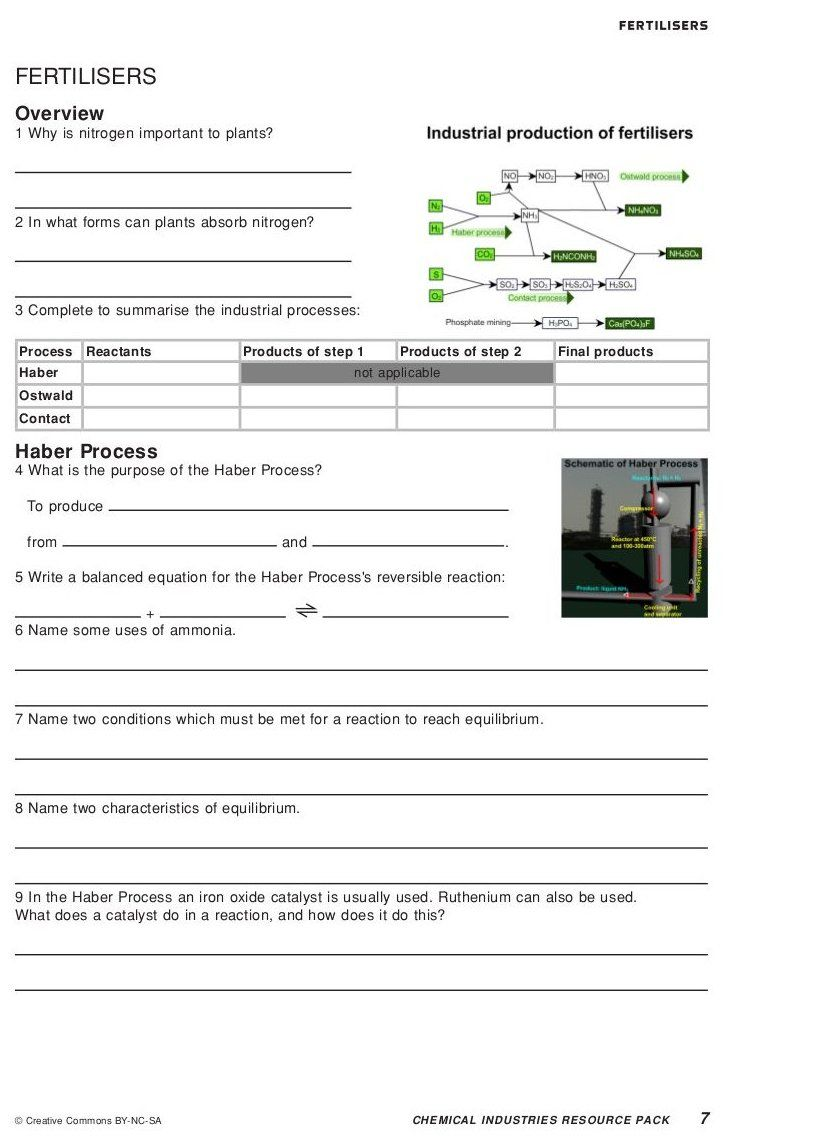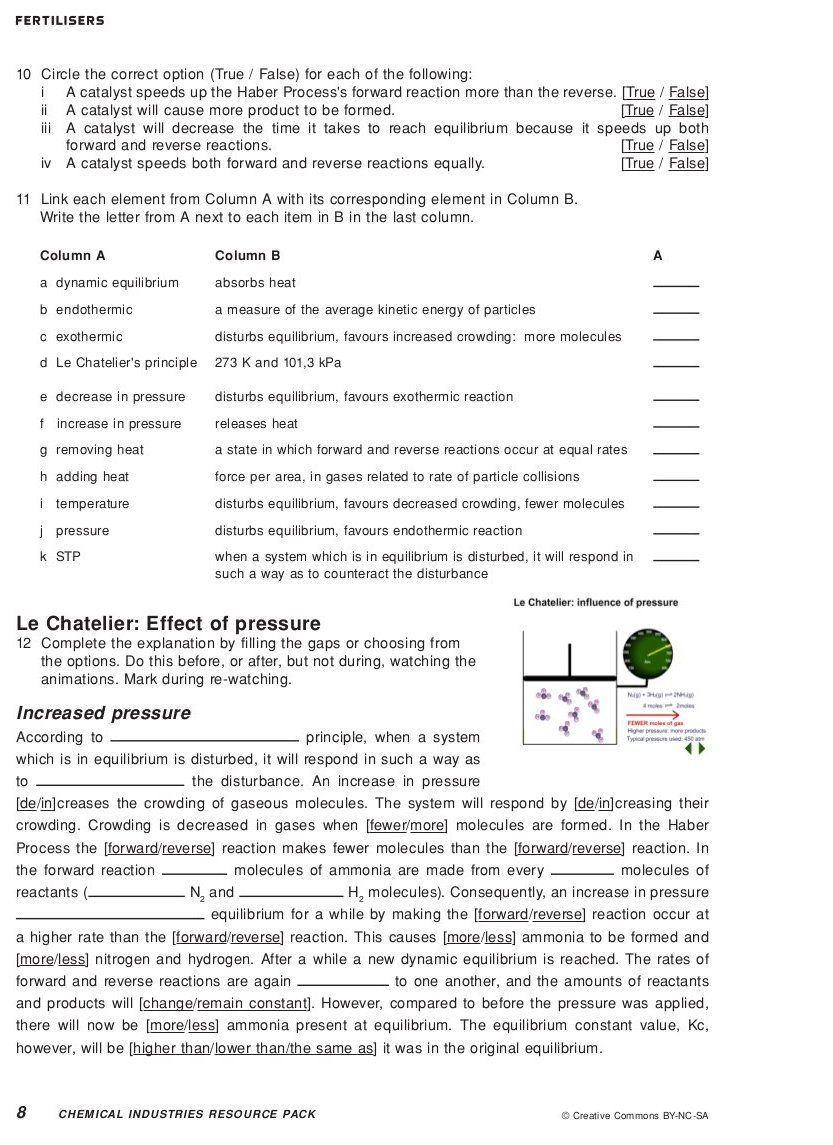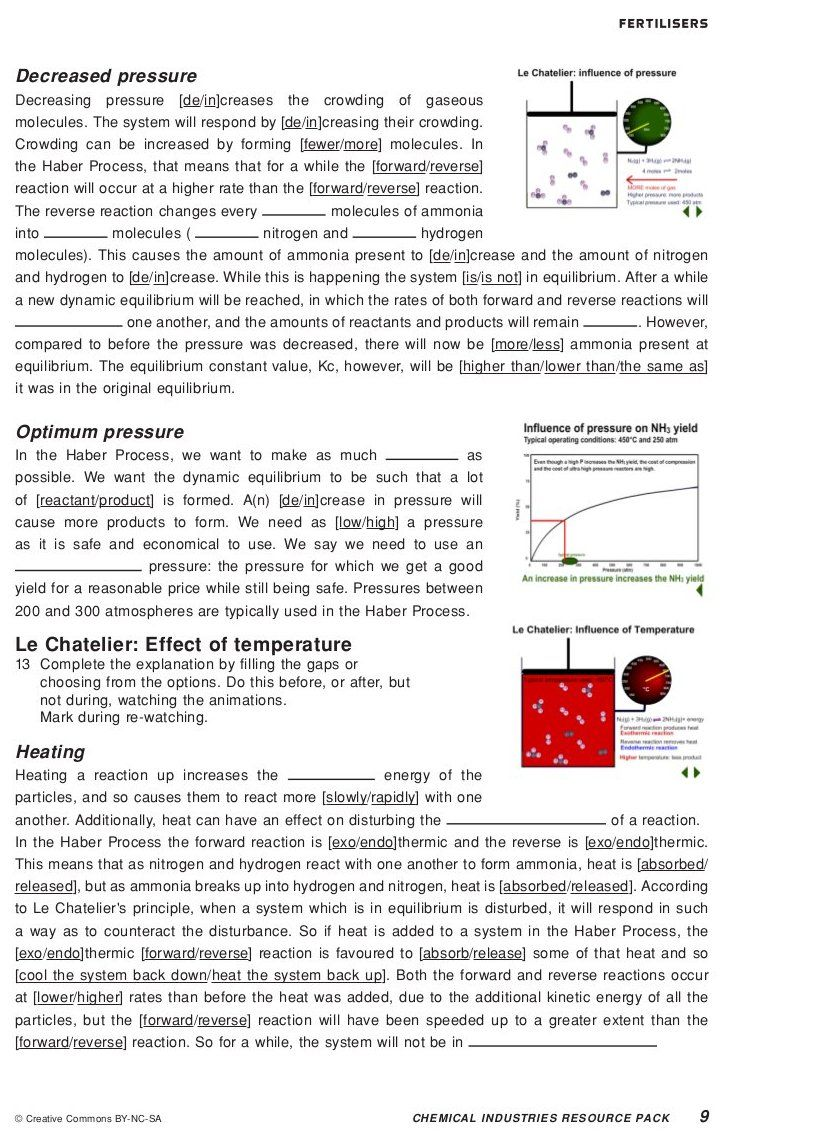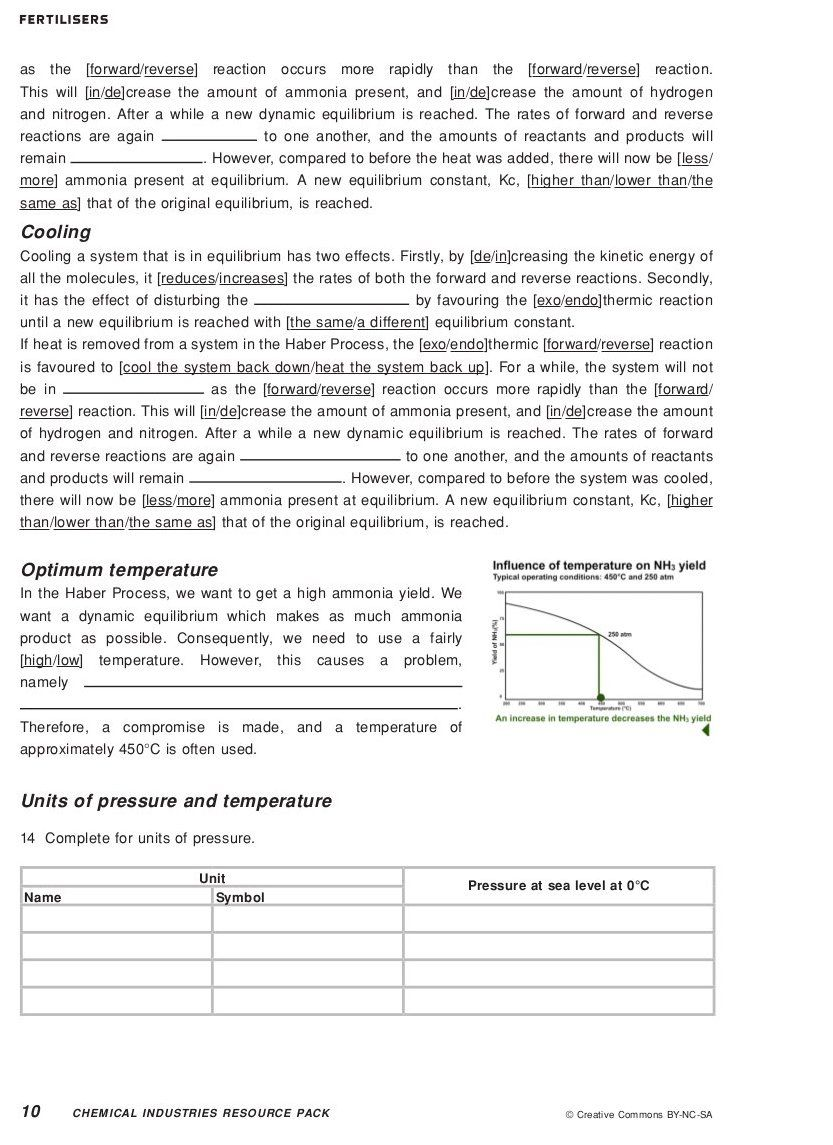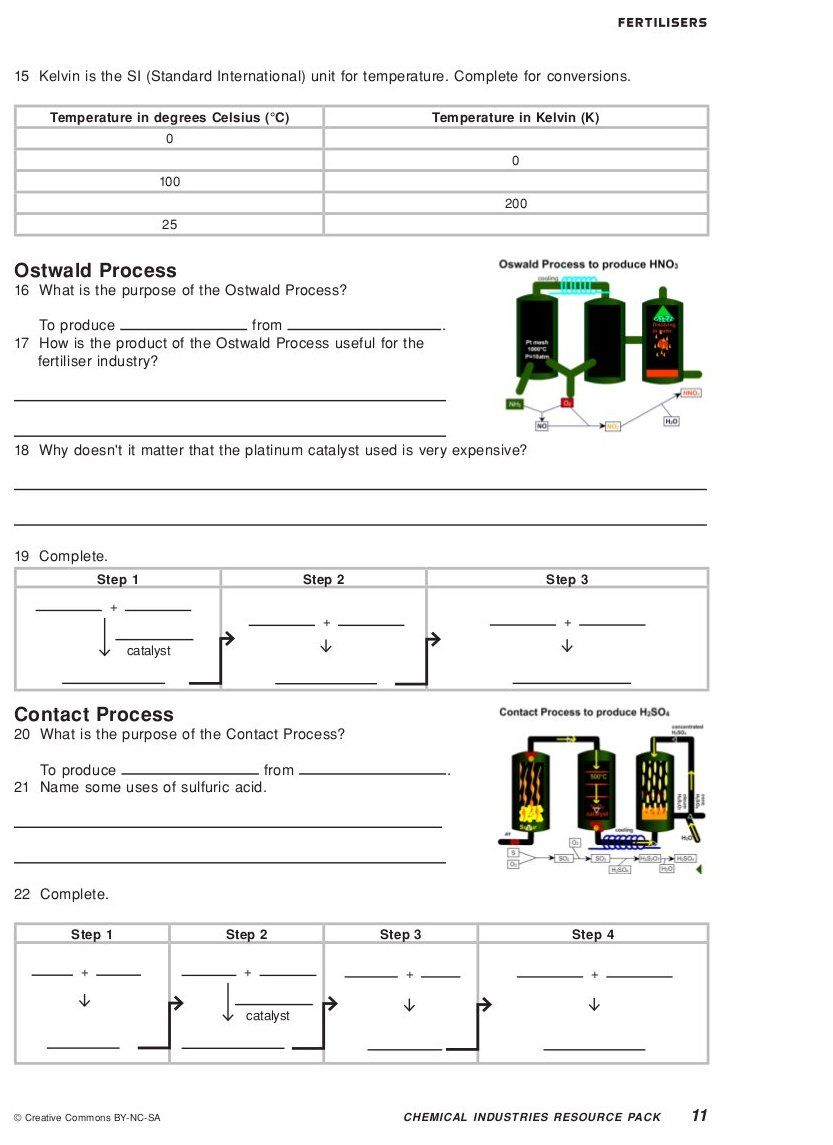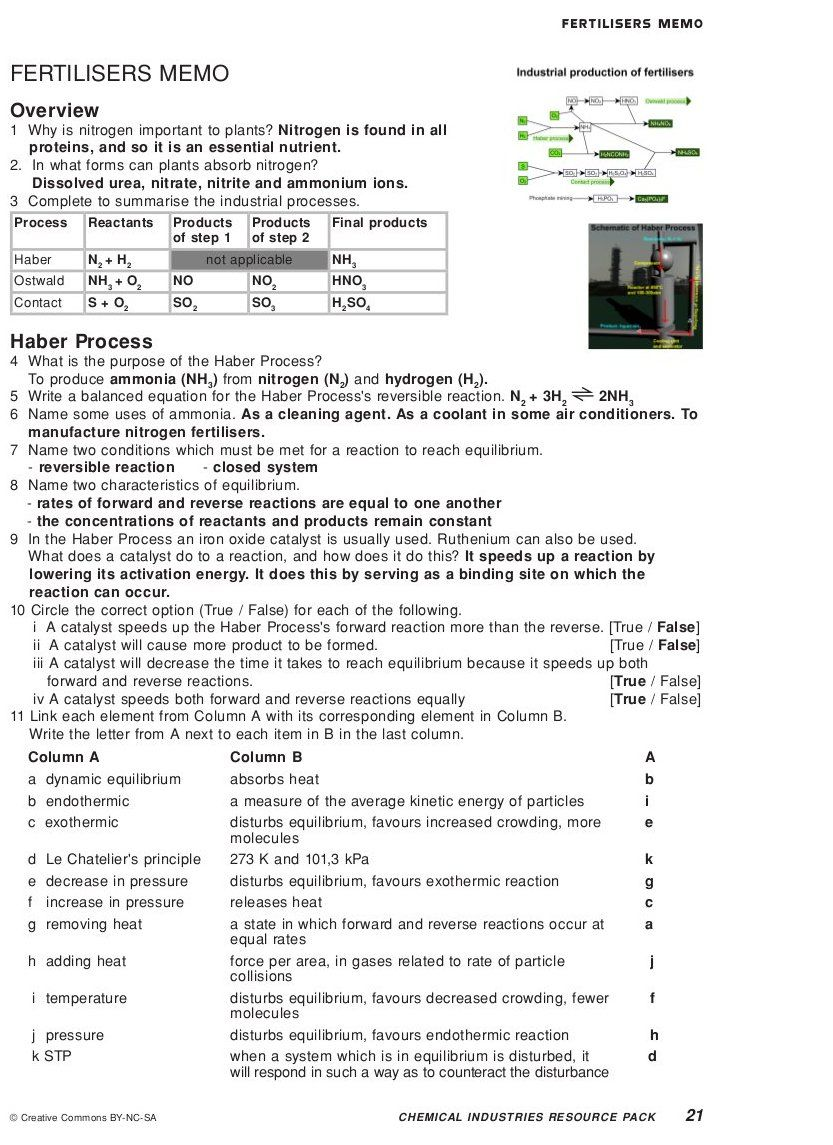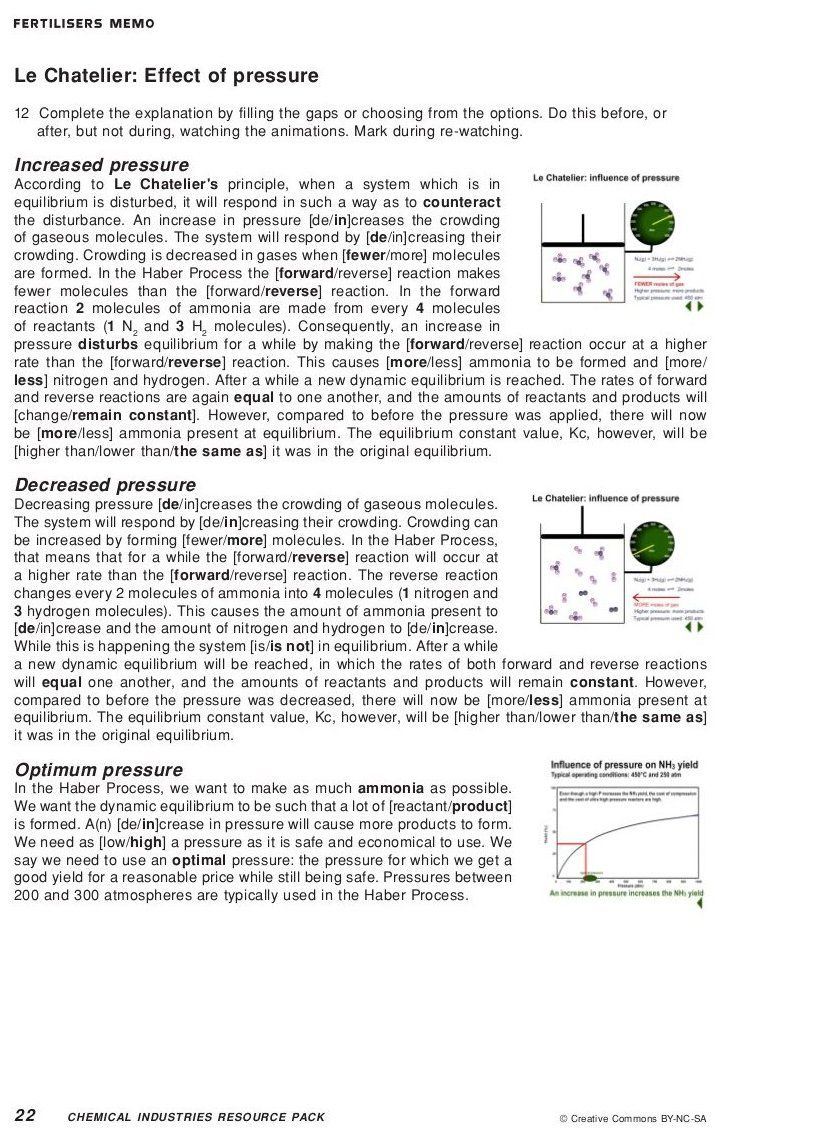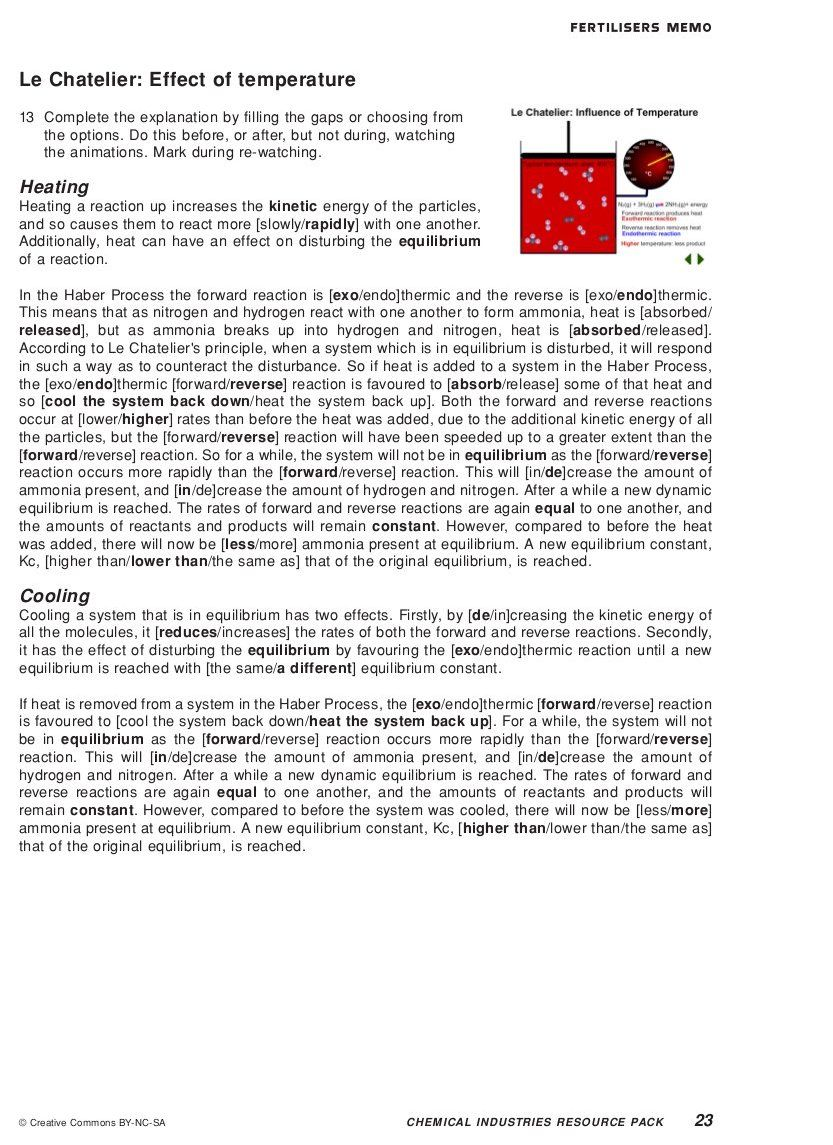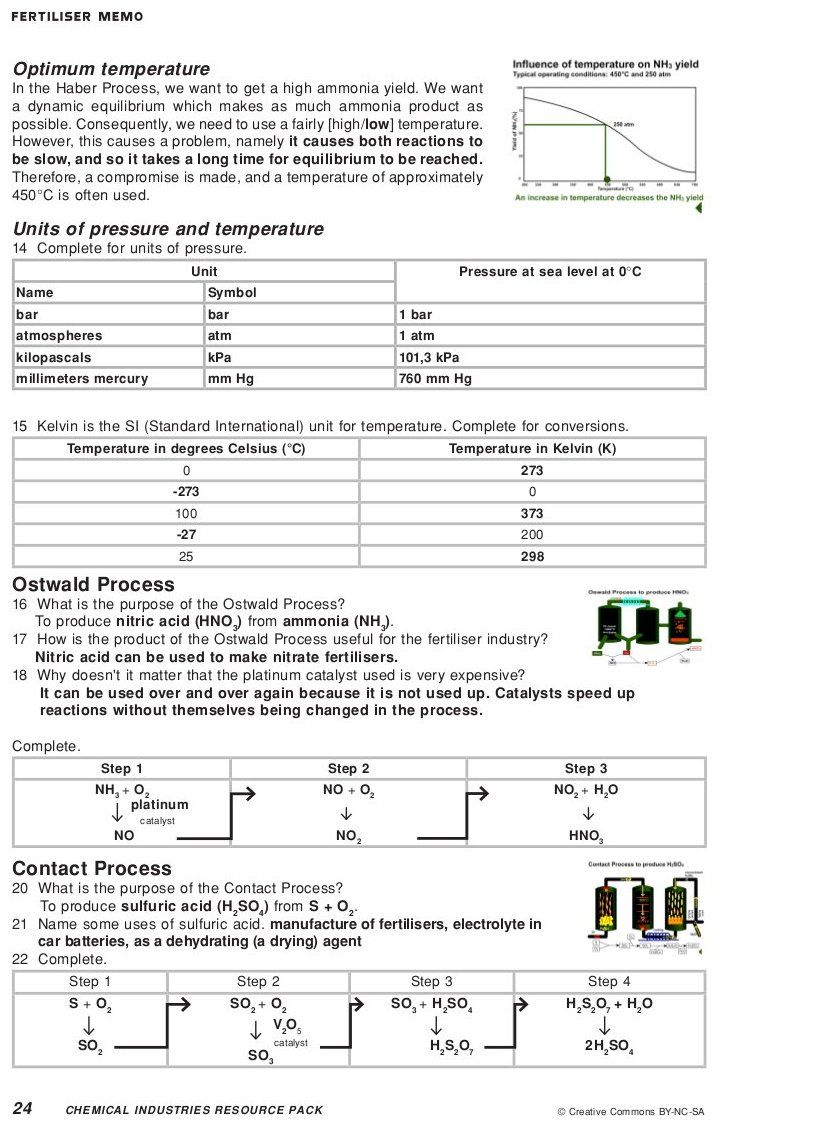## The industrial production of fertilisers

Now that you have gone through this section, watch the following animation and go through the worksheet that accompanies it.

Reproductions of the worksheets are given in the teachers guide.

## The industrial production of fertilisers

Textbook Exercise 14.2

Use the following processes to create your own mind map of the manufacturing of fertilisers:

• Fractional distillation

• Steam reforming

• Haber process

• Contact process

• Ostwald process

• you can, and should, add to this list

Include what the reactants and the products are for each process and how these link with the other processes involved.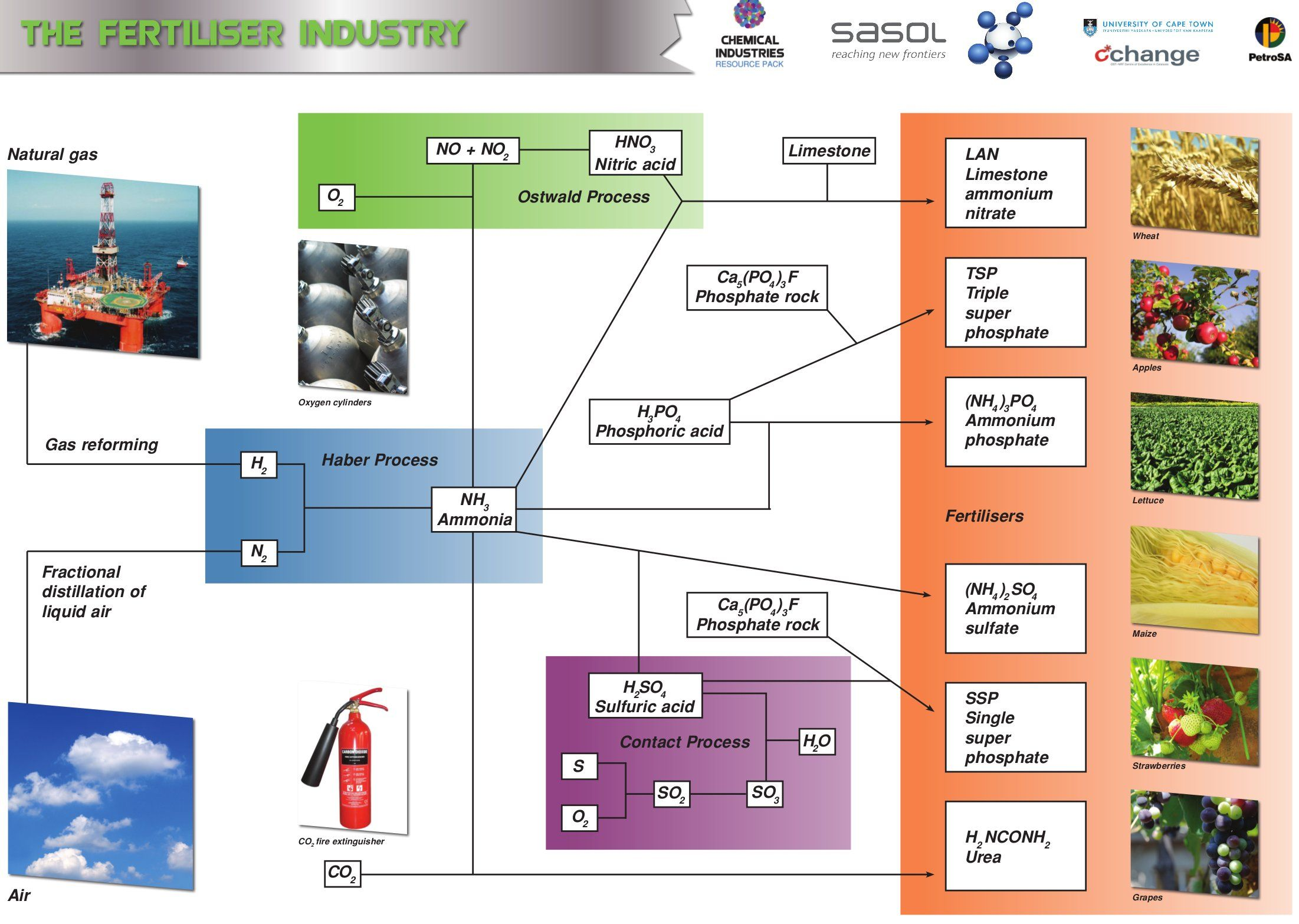Research the process of fractional distillation (from old school science textbooks, from your teacher, or the internet) and write a paragraph on how this process works.

Fractional distillation is a separation method that uses the difference in boiling temperatures of the components of a mixture to separate those components. The mixture is heated to convert the components into the vapour (gas) phase. The vapour mixture is then pumped into a tall separation column (called a fractional distillation column), usually at the bottom of the column. As the vapour mixture moves up the column and cools, the different components (called fractions) condense as the temperature drops below the various boiling point temperatures. The fractions are collected using collection trays. The fractions can be removed from the mixture in this way and thus the components are separated. This process is used to separate the components of air or crude oil.

The reaction of hydrogen and nitrogen is an exothermic reversible reaction.

Write a balanced equation for this reaction.

$$\text{N}_{2}(\text{g}) + 3\text{H}_{2}(\text{g})$$ $$\rightleftharpoons$$ $$2\text{NH}_{3}(\text{g})$$

Use your knowledge of chemical equilibrium and explain what effect the following will have on this equilibrium reaction:

raising the temperature

When the temperature of any reaction is increased, the rate of both the forward and the reverse reactions will be increased. However, the forward reaction is exothermic. Raising the temperature would favour the reaction which would remove the excess heat. The reverse reaction will therefore be favoured more than the forward reaction. This will result in more $$\text{NH}_{3}$$ decomposing to form $$\text{H}_{2}$$ and $$\text{N}_{2}$$. The overall result will be that more reactants will be formed.

raising the pressure

Raising the pressure will shift the equilibrium to decrease the pressure. This would favour the reaction which produced fewer molecules. The forward reaction produces 2 moles of $$\text{NH}_{3}$$ (compared to the 4 moles of the reverse reaction). The forward reaction will therefore be favoured. The overall result will be that more products will be formed.

Ammonia and nitric acid react to form the compound A. Compound A is soluble in water and can be used as a fertiliser.

Write down the name and formula for compound A.

ammonium nitrate, $$\text{NH}_{4}\text{NO}_{3}$$

What type of reaction takes place to form compound A.

Acid-base reaction

Write a balanced equation for the reaction to form compound A.

$$\text{NH}_{3}(\text{g}) + \text{HNO}_{3}(\text{ℓ})$$ $$\to$$ $$\text{NH}_{4}\text{NO}_{3}(\text{s})$$

Compound A is dissolved in water. Write a balanced ionic equation for this dissolution reaction. Include the state symbols in your equation.

$$\text{NH}_{4}\text{NO}_{3}(\text{s})$$ $$\to$$ $$\text{NH}_{4}^{+}(\text{aq}) + \text{NO}_{3}^{-}(\text{aq})$$

Why is it important for fertilisers to be soluble in water?

So that the plants can absorb them from the soil.

Compound A can also be used as an explosive. What precautions need to be taken when this compound is packaged and transported?

Packages need to be kept away from heat and flames.

The following reaction can be used to manufacture hydrogen gas. It is an exothermic redox reaction.

$$\text{CO}(\text{g}) + \text{H}_{2}\text{O}(\text{g})$$ $$\rightleftharpoons$$ $$\text{CO}_{2}(\text{g}) + \text{H}_{2}(\text{g})$$

Write down the reduction half reaction for this reaction.

Reduction is gain of electrons. $$\text{H}^{+}$$ in $$\text{H}_{2}\text{O}$$ gains an electron to become $$\text{H}$$.

$$2\text{H}^{+} + 2\text{e}^{-}$$ $$\to$$ $$\text{H}_{2}$$

Which compound is the reducing agent?

Carbon monoxide or $$\text{CO}$$. $$\text{CO}$$ causes $$\text{H}_{2}\text{O}$$ to be reduced.

Which compound is the oxidising agent?

Water or $$\text{H}_{2}\text{O}$$. $$\text{H}_{2}\text{O}$$ causes $$\text{CO}$$ to be oxidised.

Identify two conditions that will ensure a high yield of hydrogen.

Remove $$\text{CO}_{2}$$ and $$\text{H}_{2}$$ (the products) from the reaction vessel. More product will therefore be formed from the same amount of reactants.

Decrease the temperature. This will favour the forward (exothermic) reaction and more product will be formed with the same amount of reactants, meaning an increased yield.

(Increasing the concentration of $$\text{CO}$$ or $$\text{H}_{2}\text{O}$$ (steam) (the reactants) will increase the amount of product formed, but not the yield. More reactants means that more product must be formed to get the same yield because the maximum yield possible has increased).